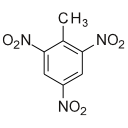Subsections

## structure.pca### Synopsis

Principle component analysis (PCA) of the motions in an ensemble of structures.

### Defaults

structure.pca(pipes=None, models=None, molecules=None, obs_pipes=None, obs_models=None, obs_molecules=None, atom_id=None, algorithm=`eigen', num_modes=4, format=`grace', dir=None)

### Keyword arguments

pipes: The data pipes to perform the PC analysis on.

models: The list of models for each data pipe to perform the PC analysis on. The number of elements must match the pipes argument. If no models are given, then all will be used.

molecules: The list of molecules for each data pipe to perform the PC analysis on. The PCA will only be calculated for atoms with identical residue name and number and atom name. The number of elements must match the pipes argument. If no molecules are given, then all will be used.

obs_pipes: The data pipes in the PC analysis which will have zero weight. These structures are for comparison.

obs_models: The list of models for each data pipe in the PC analysis which will have zero weight. These structures are for comparison. The number of elements must match the pipes argument. If no models are given, then all will be used.

obs_molecules: The list of molecules for each data pipe in the PC analysis which will have zero weight. These structures are for comparison. The PCA will only be calculated for atoms with identical residue name and number and atom name. The number of elements must match the pipes argument. If no molecules are given, then all will be used.

atom_id: The atom identification string of the coordinates of interest.

algorithm: The PCA algorithm used to find the principle components of. This can be either `eigen' for an eigenvalue/eigenvector decomposition, or `svd' for a singular value decomposition.

num_modes: The number of PCA modes to calculate.

format: The format of the plot data.

dir: The directory to save the graphs into.

### Description

Perform a principle component analysis (PCA) for all the chosen structures. 2D graphs of the PC projections will be generated and placed in the specified directory.

Support for multiple structures is provided by the data pipes, model numbers and molecule names arguments. Each data pipe, model and molecule combination will be treated as a separate structure. As only atomic coordinates with the same residue name and number and atom name will be assembled, structures with slightly different atomic structures can be compared. If the list of models is not supplied, then all models of all data pipes will be used. If the optional molecules list is supplied, each molecule in the list will be considered as a separate structure for comparison between each other.

A subset of the structures can be set as `observing'. This means that they will have a weight of zero when constructing the covariance matrix and determining its eigenvectors. Therefore the structures will not contribute to the principle components, but will be present and compared to structures used in the analysis.

The atom ID string, which uses the same notation as the spin ID, can be used to restrict the coordinates compared to a subset of molecules, residues, or atoms. For example to only use backbone heavy atoms in a protein, set the atom ID to `@N,C,CA,O', assuming those are the names of the atoms in the 3D structural file.

### Prompt examples

To determine the PCA modes of all models in the current data pipe, simply type:

```[numbers=none]
relax> structure.pca()
```

The relax user manual (PDF), created 2020-08-26.